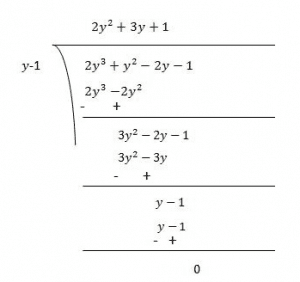Guru

# Factorize:(iv) 2y3+y2–2y–1 Q.5(4)

• 0

I don’t know how to solve this problem of class 9th ncert book of math of Polynomials chapter of exercise 2.4.  Give me the best way for solving the problem of this question Factorize:(iv) 2y3+y2–2y–1

Share

1. Let p(y) = 2y3+y2–2y–1

Factors = 2×(−1)= -2 are ±1 and ±2

By trial method, we find that

p(1) = 0

So, (y-1) is factor of p(y)

Now,

p(y) = 2y3+y2–2y–1

p(1) = 2(1)3+(1)2–2(1)–1

= 2+1−2

= 0

Therefore, (y-1) is the factor of p(y)Now, Dividend = Divisor × Quotient + Remainder

(y−1)(2y2+3y+1) = (y−1)(2y2+2y+y+1)

= (y−1)(2y(y+1)+1(y+1))

= (y−1)(2y+1)(y+1)

• 0